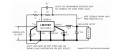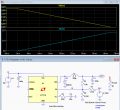# Variable output Buck regulator

#### Torbek

Joined Apr 19, 2019
45
Hi folks so I would like to produce a variable output from the following CCT. I wonder if putting a FET in to the feedback circuit and using a PIC, maybe either the simple d/a convertor or some resistors on output pins would do it. However, I don't really work with FETS so could do with advice...The module is one I have purchased from Ebay and uses a pot instead of R1&2. I would exchange R2 for a FET.

#### crutschow

Joined Mar 14, 2008
27,712
Here's the LTspice simulation of a similar buck regulator using a DAC input to control the output voltage (discussion here).

The output voltage can be adjusted from 0V to the maximum.
Note that the output voltage is inverted from the control voltage, i.e. the maximum regulator output occurs when the DAC output is zero.
You could invert that, if desired, by adding an inverting, unity-gain differential op amp configuration at the DAC output (with suitable DC offset to get 0V output with the maximum DAC voltage in).

An easy way to calculate the resistor values is to start at the half voltage point, where the DAC voltage equals the FB voltage of 2.2V (that's 1.235V for the LM2596).
Since, at the point there's no current going through R3, you can remove it from the circuit, and calculate the values of R1 and R2 to give the desired 1/2 full-scale output voltage (in this case 10V).
Then you set the DAC voltage to 0V which effectively puts R3 in parallel with R2, and calculate the value of R3 to give the desired full-scale output voltage (here 20V).
These resistor values also mean that a voltage of double the FB voltage (4.4V) from the DAC will generate 0V at the converter output.

Using a FET for this purpose would be problematic because of the nonlinear relation between the FET's gate-source voltage and its on-resistance.Last edited:
•Delta Prime, Irving and bertus